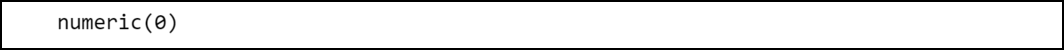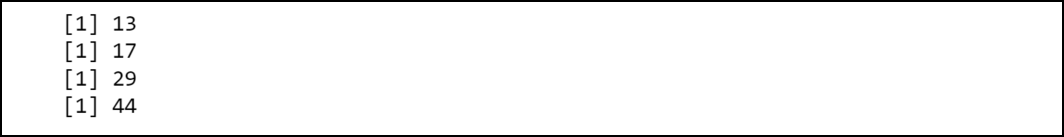GFG App
Open AppBrowser
Continue

# How to Fix in R: Replacement has length zero

In this article, we will discuss how to fix the error of replacement has length zero in the R programming language.

## Replacement has length zero:

The R compiler produces such an error. Generally, this error takes the below form:

`Error in vect : replacement has length zero`

The compiler produces this error when a programmer tries to replace a value in a vector with some other value but the other value has a length equal to zero which implies that the other value has no existence.

## When this error might occur:

Consider an example in which we have a vector initialized with 5 five values.

## R

 `# Initializing a vector ` `vect = ``c``(5, 8, 4, 12, 15)`

Now suppose we want to iterate over the vector and at each step of the iteration we want to assign the sum of the current value and the value stored at the previous position at the current position.

## R

 `# Initializing a vector ` `vect = ``c``(5, 8, 4, 12, 15) ` ` `  `# Iterate over the vector ` `for ``(i ``in` `1 : ``length``(vect)) { ` `   `  `  ``# Assign sum ` `  ``vect[i] = vect[i] + vect[i - 1] ` `}`

Output:Output

The R compiler produces this error because of the case:

`vect = vect + vect`

This is because indexing in R begins from 1. Hence, vect doesn’t exist.

We can confirm that vect doesn’t exist by simply printing the value:

Example:

## R

 `# Print the value stored at the index 0 ` `print``(vect `

Output:Output

The output is a numeric vector having a length equal to zero.

## Fixing the error:

We can fix this error by simply taking care of the case that may access the value that doesn’t exist during the iteration.

Example:

## R

 `# Initializing a vector ` `vect = ``c``(5, 8, 4, 12, 15) ` ` `  `# Iterate over the vector ` `for ``(i ``in` `2 : ``length``(vect)) { ` `   `  `      ``# Assign sum ` `    ``vect[i] = vect[i] + vect[i - 1] ` `     `  `    ``# Print the value ` `    ``print``(vect[i]) ` `} `

Output:Output

My Personal Notes arrow_drop_up